﻿ 低频天气图在四川盆地强降水预报的应用研究 Application of Low Frequency of Heavy Precipitation Forecast Weather Map in the Sichuan Basin

Vol.08 No.03(2018), Article ID:25590,15 pages
10.12677/AG.2018.83066

Application of Low Frequency of Heavy Precipitation Forecast Weather Map in the Sichuan Basin

Yang Liu, Tiangui Xiao, Peirong Li

Atmospheric Science College, Chengdu University of Information Technology, Chengdu Sichuan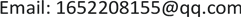Received: Jun. 7th, 2018; accepted: Jun. 20th, 2018; published: Jun. 27th, 2018ABSTRACT

This paper used the 500 hPa wind data in the summer form 2013 to 2017 and daily precipitation data of 132 stations of Sichuan basin to draw a low-frequency weather map and determine eight key areas according to geographical location of the Sichuan Basin. Then we analyzed the relation of low frequency system and summer precipitation by using the Low-frequency weather chart method and established a forecast model for Sichuan Basin summer heavy precipitation. We did a test of heavy precipitation forecast in Sichuan Basin by this method, and we found that there are many empty forecasts. Therefore, we must revise the advanced analytic result of forecast model to improve the accuracy of forecasts greatly. After comparing the results of forecast test with the actual results, we found that the effect by using the low-frequency weather map method for to forecast heavy precipitation in June is much better.

Keywords:Sichuan Basin, Low Frequency Synoptic Map, Low Frequency Key Zones, Forecast Model, Forecast Test1. 引言

20世纪70年代初热带大气季节内震荡由Madden and Julian (1971; 1972)发现，后来以Madden和Julian的名字被称为Madden Julian Oscillation，是目前全球发现的最强低频信号   。目前的研究成果是关于大气低频的研究主要集中在它的活动及其对热带的异常现象以及对全球气候系统的产生的影响，它的数值模拟(预报)研究，以及与厄尔尼诺与南方涛动事件的相互联系这三个方面  。由于热带MJO的准周期性、与热带对流的密切相关性以及其时间尺度与延伸期预报时效的匹配性，使得MJO成为了目前延伸期天气预报的重要桥梁  。当前针对热带MJO的研究，是用于延伸期预报，针对热带MJO的延伸期预报运用的是统计模型和动力模式两种方法，统计模型主要利用滞后回归模型、自回归模型、组合相似法和经验位相传播     等方法来实时预报MJO，也有在多种统计方法相结合的基础上对MJO进行集合预报，如韩国利用三种统计学的理论即小波的周期分析、多元线性回归和奇异谱分析相结合的方式来预报对MJO，再与统计预报相结合，预报时效可以达到24天  。

2. 资料和方法

2.1. 资料概况

1) 四川2013~2017年5~8月逐日NCEP再分析数据，水平分辨率为2.5˚ × 2.5˚，垂直方向为，海平面，500 hPa，要素场主要包括U风场、V风场，通过处理将nc数据转换为dat格式，区域范围为0˚~70˚N，60˚~150˚E。

2) 2013年~2017年四川省155个站的逐日降水量。因为数据原因，最终选取132个站点，四川盆地132个站点分布图如图1所示。

2.2. 方法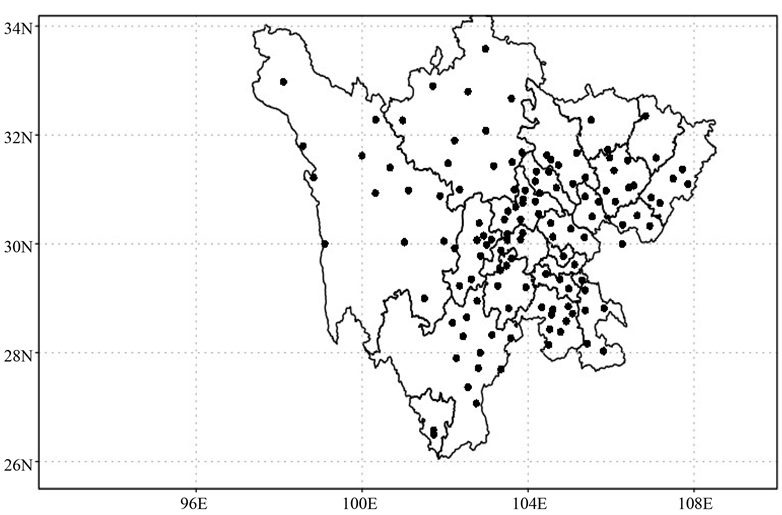Figure 1. 132 stations in the Sichuan Basin

3. 低频天气图特征

3.1. 低频天气图的建立

${U}_{n}={a}_{0}\left({{U}^{\prime }}_{n}-{{U}^{\prime }}_{n-2}\right)-{b}_{1}{U}_{n-1}-{b}_{2}{U}_{n-2},\text{\hspace{0.17em}}\text{\hspace{0.17em}}n=1,2,\cdots ,N$

${a}_{0}=\frac{2\Delta \Omega }{4+2\Delta \Omega +{\Omega }_{0}^{2}},{b}_{1}=\frac{2\left({\Omega }_{0}^{2}-4\right)}{4+2\Delta \Omega +{\Omega }_{0}^{2}},{b}_{2}=\frac{4-2\Delta \Omega +{\Omega }_{0}^{2}}{4+2\Delta \Omega +{\Omega }_{0}^{2}}$

$\begin{array}{l}\Delta \Omega =2|\frac{\mathrm{sin}\left({\omega }_{1}\Delta t\right)}{1+\mathrm{cos}\left({\omega }_{1}\Delta t\right)}-\frac{\mathrm{sin}\left({\omega }_{2}\Delta t\right)}{1+\mathrm{cos}\left({\omega }_{2}\Delta t\right)}|\\ {\Omega }_{0}=\frac{4\mathrm{sin}\left({\omega }_{1}\Delta t\right)\mathrm{sin}\left({\omega }_{2}\Delta t\right)}{\left(1+\mathrm{cos}\left({\omega }_{1}\Delta t\right)\right)\left(1+\mathrm{cos}\left({\omega }_{2}\Delta t\right)\right)}\end{array}$

$\Delta t=1\left(\text{d}\right),\text{\hspace{0.17em}}{\omega }_{0}=\text{2π}/{m}_{2},\text{\hspace{0.17em}}{\omega }_{1}=\text{2π}/{m}_{1},\text{\hspace{0.17em}}{\omega }_{2}={\omega }_{0}^{2}/{\omega }_{1}$

3.2. 低频天气系统活动周期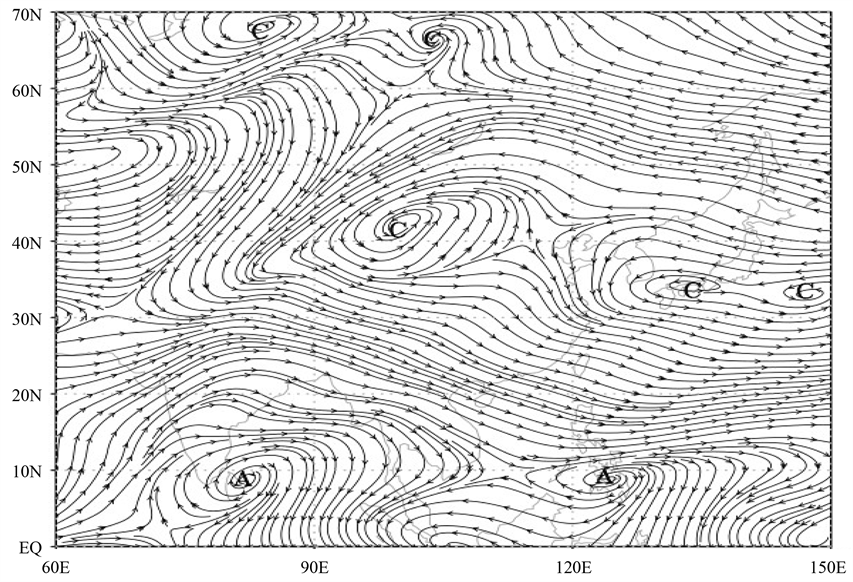Figure 2. 500 hPa low-frequency weather map (July 4, 2017) (A: low-frequency anticyclone, C: low-frequency cyclone)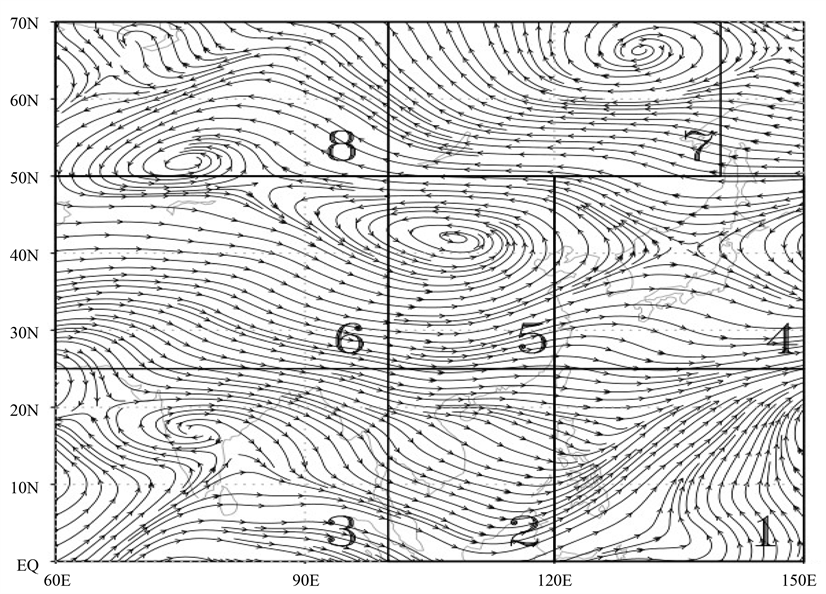Figure 3. Low frequency weather system critical area

3.3. 低频天气系统变化路径

3.4. 低频天气系统与强降水的配置Table 1. Low-frequency synoptic oscillation period in key areas (unit: days)

4. 低频天气图在四川盆地的应用

4.1. 建立四川盆地夏季强降水低频预报模型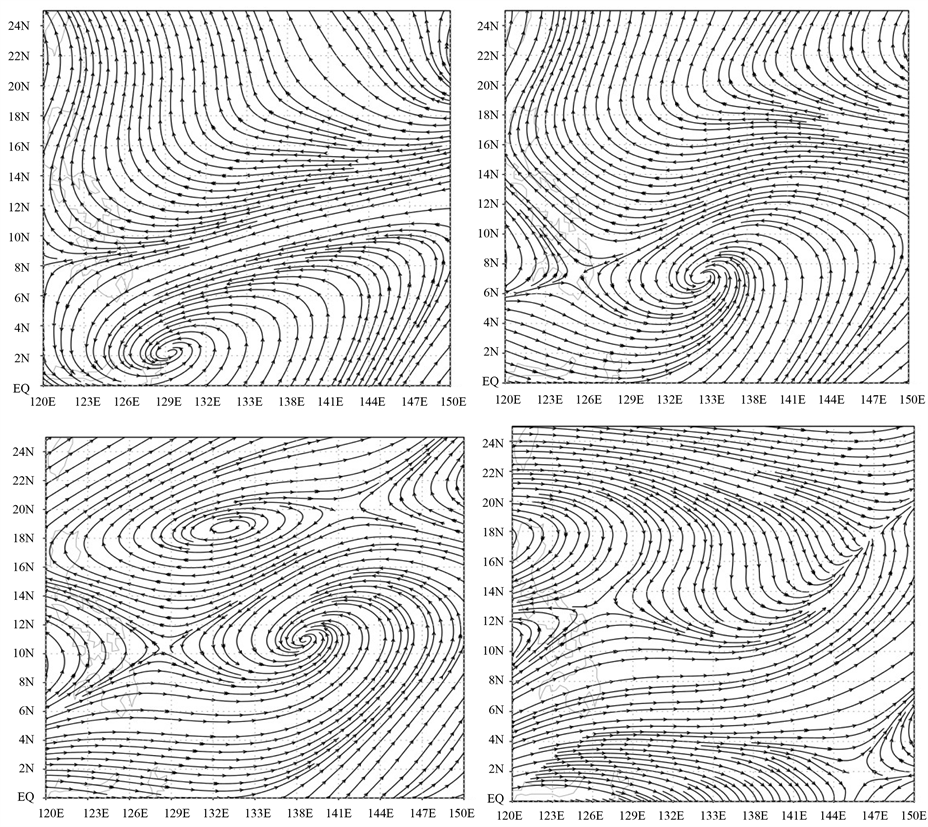Figure 4. Mobile change path

4.2. 强降水过程的预报流程

4.3. 2017年四川夏季强降水过程预报应用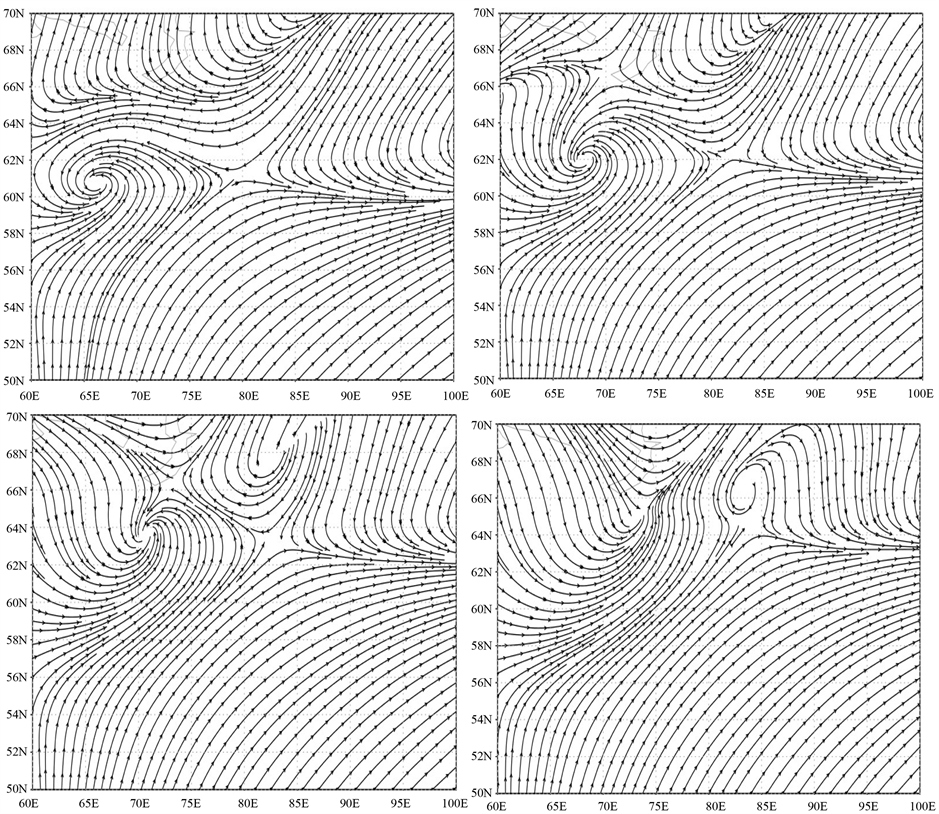Figure 5. Oscillatory change path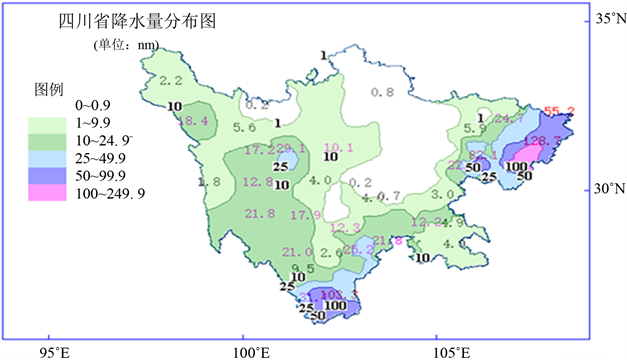Figure 6. Sichuan Province, July 7, 2017 heavy precipitation daily precipitation distribution map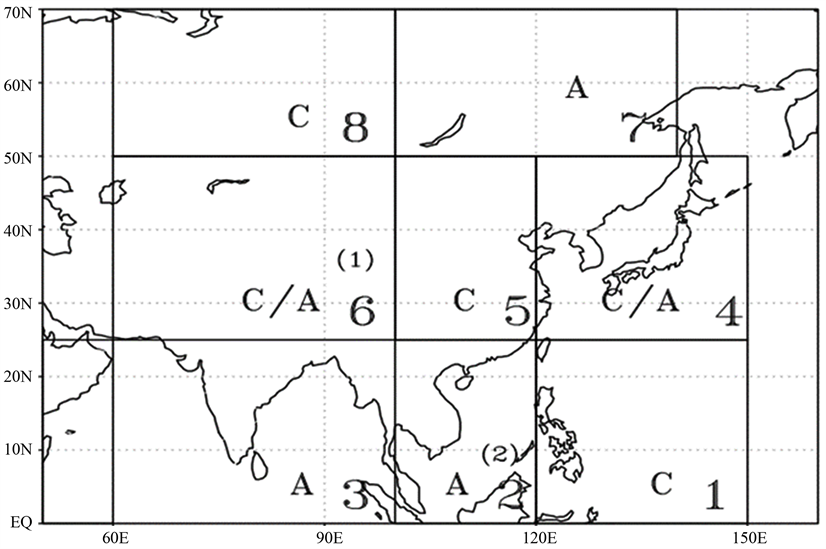Figure 7. Low-frequency prediction model of heavy precipitation in Sichuan Basin (C: low-frequency cyclone, A: low-frequency anticyclone, arrow (1): cold air in the north, arrow (2): warm south air in the south)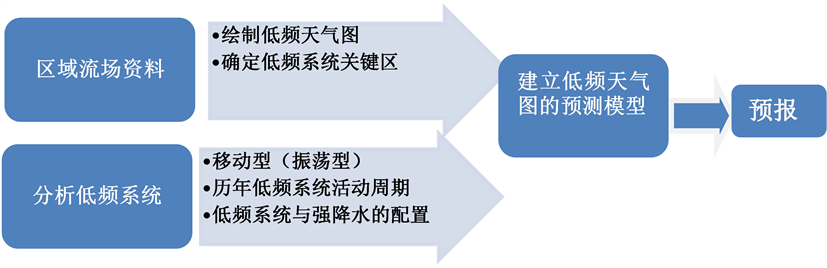Figure 8. Forecast process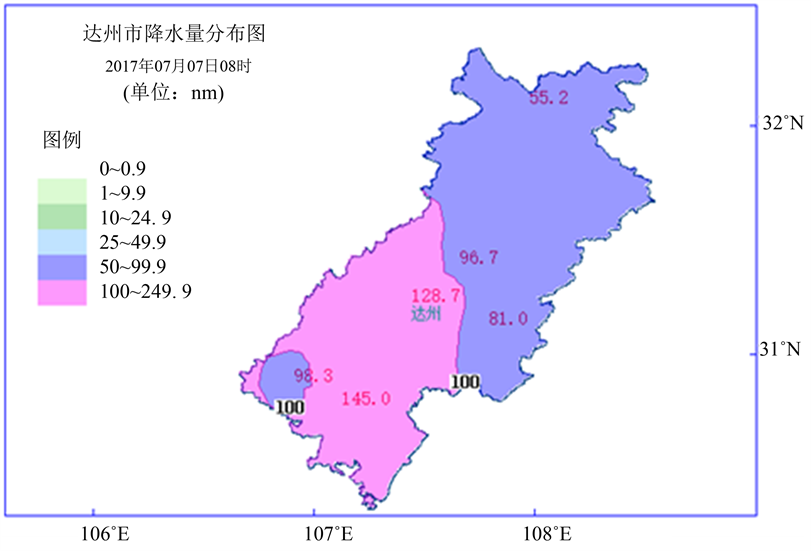Figure 9. Precipitation in Rida Prefecture with heavy precipitationTable 2. Prediction of heavy precipitation process in Sichuan Basin from June to July 2017

4.4. 低频天气图的天气学意义

4.5. 完善技术

1) 低频天气图方法有很明显的地域性，适合在区省、市的气象单位进行应用推广。但应用时，需要着重考虑地域的大气环流、天气系统、地形地理等方面的特点，把这些特点加在低频天气图方法中，就会得到比较有地域性的低频天气图方法。

2) 低频天气图方法要以天气动力学原理为基础，把实际天气图(未滤波)上的高频变化的天气系统滤去，改为具有缓变特征的低频天气图上的低频系统。

3) 低频天气图方法预报流程中，有几个预报流程(关键区的选择、低频图的分析和预测模型的建立) 非常重要。如分析低频天气图，作为预报人员要对天气学原理和技术规则十分了解，能够读懂低频天气图，了解低频系统的演变特征，这是低频天气图方法的基本功。

5. 结论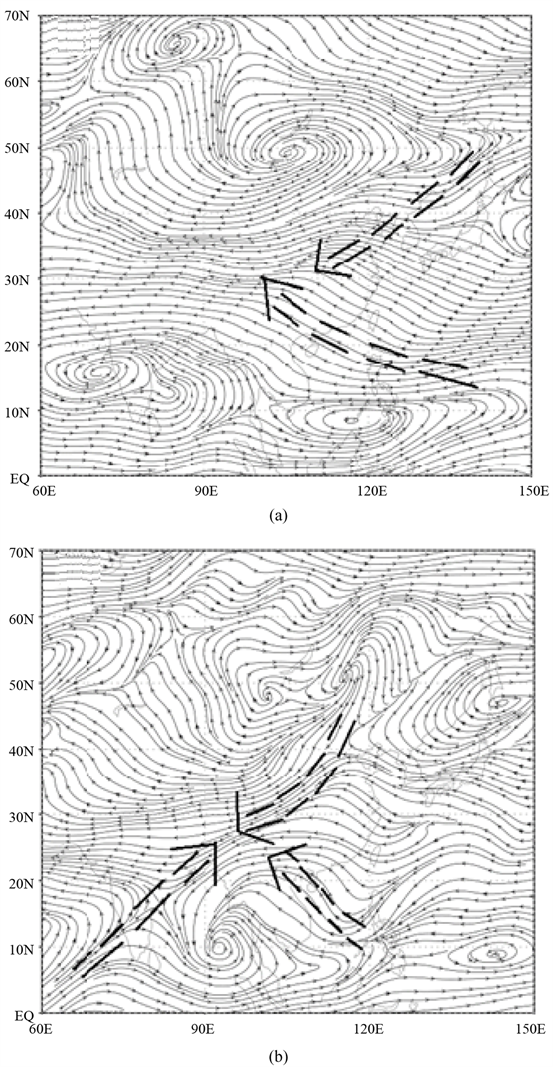Figure 10. June 23, 2017 Flow Field Map ((a) Low Frequency Flow Field Diagram, (b) Live Flow Field Diagram)

Application of Low Frequency of Heavy Precipitation Forecast Weather Map in the Sichuan Basin[J]. 地球科学前沿, 2018, 08(03): 618-632. https://doi.org/10.12677/AG.2018.83066

1. 1. Blackmon, M.L., Wallace, J.M., Lau, N.C., et al. (1977) An Observational Study of the Northern Hemisphere Wintertime Circulation. Journal of the Atmospheric Sciences, 34, 1040-1053. 2.0.CO;2>https://doi.org/10.1175/1520-0469(1977)034<1040:AOSOTN>2.0.CO;2

2. 2. Hoskins, B.J. and Pearce, R. (1983) Large Scale Dynamical Process in the Atmosphere. Academic Press, Reading, 56-65.

3. 3. 李崇银, 潘静, 宋洁. MJO研究新进展[J]. 大气科学, 2013(2): 229-252.

4. 4. 梁萍, 何金海. MJO在延伸期预报中的应用进展[J]. 气象科技进展, 2013(1): 31-38.

5. 5. Maharaj, E.A. and Wheeler, M. (2005) Forecasting an index of the Madden-Oscillation. International Journal of Climatology, 25, 1611-1618. https://doi.org/10.1002/joc.1206

6. 6. Jiang, X.N., Waliser, D.E., Wheeler, M.C., et al. (2008) Assessing the Skill of an All-Season Statistical Forecast Model for the Madden-Julian Oscillation. Monthly Weather Review, 136, 1940-1956. https://doi.org/10.1175/2007MWR2305.1

7. 7. Jones, C., Carvalho, L.M.V., Higgins, R.W., et al. (2004) A Statistical Forecast Model of Tropical Intraseasonal Convective Anomalies. Journal of Climate, 17, 2078-2095. https://doi.org/10.1175/1520-0442(2004)017<2078:ASFMOT>2.0.CO;2

8. 8. Seo, K., Wang, W., Gottschalk, J., et al. (2009) Evaluation of MJO Forecast Skill from Several Statistical and Dynamical Forecast Models. Journal of Climate, 22, 2372-2388. https://doi.org/10.1175/2008JCLI2421.1

9. 9. Kang, I.S. and Kim, H.M. (2011) Intraseasonal Prediction and Predictability for Boreal Winter. In: Chang, C.P., et al., Eds, The Global Monsoon System: Research and Forecast, World Scientific Series on Asia-Pacific Weather and Climate, Vol 5. World Scientific, Singapore, 409-418. https://doi.org/10.1142/9789814343411_0024

10. 10. 孙国武, 信飞, 陈伯明, 等. 低频天气图预报方法[J]. 高原气象, 2008(S1).

11. 11. 梁萍, 丁一汇. 基于季节内振荡的延伸预报试验[J]. 大气科学, 2012, 36(1): 102-116.

12. 12. 陈丽娟, 陈伯民, 张培群, 等. T63模式月动力延伸预报高度场的改进实验[J]. 应用气象学报, 2005, 16(增刊): 92-96.

13. 13. 覃志年, 李维京, 何慧, 等. 广西6 月区域性暴雨过程的延伸预测试验[J]. 高原气象, 2009, 28(3): 668-693.

14. 14. Sun, G. and Chen, B. (1989) Oscillation Characteristics and Meridional Propagation of Atmospheric Low Frequency Waves over Qinghai-Xizang Plateau. Chinese Journal of Atmospheric Sciences, 12, 293-300.

15. 15. 章基嘉, 孙国武, 陈葆德. 青藏高原大气低频变化的研究[M]. 北京: 气象出版社, 1991: 105-106.

16. 16. 杨玮, 何金海, 孙国武, 等. 低频环流系统的一种统计预报方法[J]. 气象与环境学报, 2011, 27(3): 1-5.

17. 17. 蒋薇, 孙国武, 陈伯民, 等. 江苏省汛期强降水过程的延伸期预报试验[J]. 气象科学, 2011, 31(增刊): 24-30.

18. 18. 胡春丽, 李辑, 陈伯民, 等． 低频天气图方法在辽宁夏季延伸期强降水预报中的应用[J]. 气象科技进展, 2013, 3(1): 64-67.

19. 19. 孙国武, 信飞, 孔春燕, 等. 大气低频振荡与延伸期预报[J]. 高原气象, 2010, 29(5): 1142-1147.

20. 20. 张琪, 李跃清. 近48年西南地区降水量和雨日的气候变化特征[J]. 高原气象, 2014(2): 372-383.